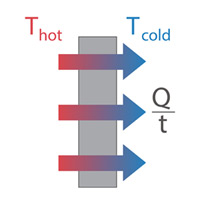Join us at the International Thermal Conductivity Conference (ITCC) and the International Thermal Expansion Symposium (ITES).

# Heat Transfer Conduction Calculator

The conduction calculator deals with the type of heat transfer between substances that are in direct contact with each other. Heat exchange by conduction can be utilized to show heat loss through a barrier. For a wall of steady thickness, the rate of heat loss is given by:

$Q = \frac{KA(T_{hot}{-}T_{cold})}{d}$

Where:
$$Q$$ = Conduction heat transfer (W)
$$K$$ = Materials thermal conductivity (W/mK)
$$A$$ = Cross sectional area ()
$$T_{Hot}$$ = Higher temperature (°C)
$$T_{Cold}$$ = Colder temperature (°C)
$$d$$ = Material thickness (m)### How does the heat transfer conduction calculator works?

The heat transfer conduction calculator below is simple to use.

1. Enter the thermal conductivity of your material (W/m•K)
2. OR select a value from our material database .
3. Input the cross-sectional area (m2)
5. Enter the hot side temperature (°C)
6. Enter the cold side temperature (°C)
7. Click “CALCULATE” solve for conduction heat transfer
 Thermal conductivity of material (W/mK) or Cross Sectional Area (m2) Thickness (m) Hot Side Temperature (°C) Cold Side Temperature (°C)
 Conduction Heat Transfer (W):